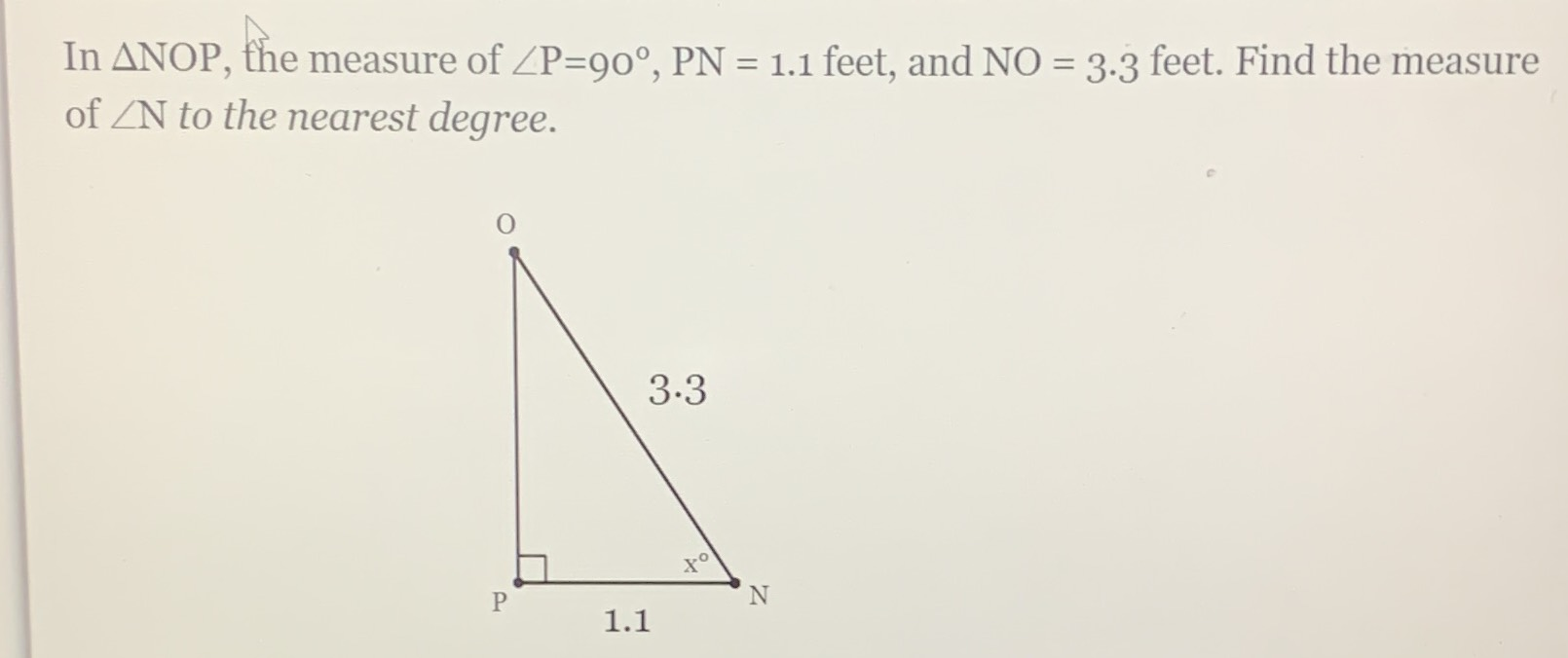Still have math questions?

Trigonometry
QuestionIn $$\triangle\mathrm{NOP}$$, the measure of $$\angle\mathrm{P}=90^{\circ}$$$$\mathrm{PN}=1.1$$, and $$\mathrm{NO}=3.3$$ feet. Find the measure of $$\angle\mathrm{N}$$ to the nearest degree.

$$\angle\mathrm{N}=71^{\circ}$$